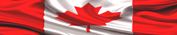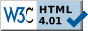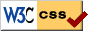HOME PAGE --------------- HELP+ » e-Design Notes --- » Switches, R, F » Resistors » Capacitors » Inductors » Diodes » Transistors --- » Potential Diff » Op Amps » Analog Filters --- » Boolean Algebra » Logic Gates » Flip-Flops » Logic ICs --- » Auto Injectors ---------------# ELECTRONIC DESIGN NOTES - RESISTORSIn Electronic Design Notes #1 was mentioned we can control the current passing through electrical circuits using resistors. Of course, there are other means to achieve good current control, but resistors are simple, cheap, and very efficient electronic components.

Control is the true magic word in electronics. No matter what we do, the purpose is to take control over voltages, currents, frequencies, and over various functions. Once we achieve total control, then we can do whatever we can think of. Resistors help us control all electrical characteristics that are calculated based on resistance: that is, current, voltage, frequency, etc.

There are a few basic things we need to know about resistors, therefore they are listed summarily as follows:1. Types of Resistors
2. Useful Formulas
3. Resistors Color Code Chart
4. Equivalent Series and Parallel Resistors
5. Controlling Voltages and Currents
6. Detecting Current Sense
7. Frequency Contro
l

NOTE

The basic notions highlighted in this page are related to a few electronic design topics presented in the first part, Hardware Design, of
LEARN HARDWARE FIRMWARE AND SOFTWARE DESIGN.

1. TYPES OF RESISTORS

There is an incredible variety of resistors, though in this page are presented only a few main groups. First of all, resistors are groped in:

A. fixed
B. variable

Depending on the way we insert them into electrical circuits, resistors can be:

a. through hole (TH)
b. surface mount (SM)
c. various mechanical fixturesFurther, resistors are built as:

1. carbon composition
2. metal film
3. carbon film
4. wirewound

Of course we could differentiate resistors based on the power (current) they can safely handle, but there are way too many types available.

Variable resistors come in a few particular types. They are important to note here because each type requires specific schematics:

1. potentiometers
2. rheostats
4. programmable potentiometers (details of hardware schematics and firmware programming source code are presented in
LEARN HARDWARE FIRMWARE AND SOFTWARE DESIGN)Fig1: Rheostat and  Potentiometer wiring    Schematics The Rheostat circuit is used to control currents (Iv) The Potentiometer circuit is used to control  voltages (Uv)2. USEFUL FORMULAS

In order to work with resistors we need a few formulas, as follows:

 RESISTORS' FORMULAS FORMULA NAME R [Ω]= ρ * L / A Resistance (details are in Design Notes 1) R [Ω] = U [V] / I [A] Ohm's Law in DC circuits (details are in Design Notes 1) Z [Ω] = U [V] / I [A] Ohm's Law in AC circuits (details are in Design Notes 1) Z = √[R2 + (XL - Xc)2] Impedance (details are in Design Notes 1) XL [Ω] =  2*PI*f [Hz]*L [H] Inductive Reactance Xc [Ω]= 1/2*PI*f [Hz]*C [C] Capacitive Reactance Rt = Ro * (1 + α * t) Rt = Resistance at current temperature Ro = resistance at 0 Celsius t = actual temperature α = temperature coefficient of resistivity G [Siemens] = 1 / R Conductance σ = 1/ρ Electrical conductivity P [W] = U [V] * I [A]   P [W] = I2[A] * R [Ω] DC power 1 [hp] = 746 [W] Conversion to horsepower 1 [kW] = 1.34 [hp] Conversion to kilowatt W [J] = U [V] * I [A] * T [s] DC Energy η = Pout / Pin Efficiency

3. RESISTORS COLOR CODE CHARTFixed resistors of the "through hole" type are marked using a special color code.

The new SM (surface mount) types are marked using numbering systems specific to each manufacturer--please consult their Data Sheets.

 RESISTOR COLOR CODE Color 1st Band 2nd Band 3rd Band Multiplier Tolerance Black 0 0 0 100 - Brown 1 1 1 101 (+/-)1% Red 2 2 2 102 (+/-)2% Orange 3 3 3 103 (+/-)3% Yellow 4 4 4 104 (+/-)4% Green 5 5 5 105 (+/-)0.5% Blue 6 6 6 106 (+/-)0.25% Violet 7 7 7 107 (+/-)0.1% Gray 8 8 8 108/10-2 - White 9 9 9 109/10-1 - Gold - - - 10-1 (+/-)5% Silver - - - 10-2 (+/-)10% None - - - - (+/-)20%

NOTE

The third color band could be missing.4. EQUIVALENT SERIES AND PARALLEL RESISTORS

The equivalent of series resistors is calculated with:          RT       =
Σ Ri
The equivalent of parallel resistors is calculated with:         1/RT =
Σ
1/Ri

Calculation examples for three resistors are presented next.Fig 2: The equivalent resistance of 3 series resistors         RT = R1 + R2 + R3         RT = 2K +3K + 4K = 9K The series equivalent RT is greater than the greatest componentFig 3: The equivalent resistance of 3 resistors in parallel         1/RT = 1/R1 +1/R2 + 1/R3         RT = R1*R2*R3 / (R2*R3 + R1*R3 +R1*R2)         RT = 24 / (12 + 8 + 6) = 24 / 26 = 0.923K The parallel equivalent RT is always smaller than the smallest component5. CONTROLLING VOLTAGES AND CURRENTS

First thing, please take a look at Figs 4 and 5.Fig 4: Voltage control circuit Voltage divider formula:  Ui = (U * Ri) / RT U = 12V = total voltage applied Ri = 3Ω = incremental resistance RT = 1Ω + 3Ω = 4Ω = total resistance Ui = (12 * 3) / 4 = 9V = incremental voltageFig 5: Current control circuit Imax = U / R1 = 12 / 3 = 4mA

In Fig 4, we control the voltage Ui using a "voltage divider" schematic. We can even adjust Ui if we use a potentiometer schematic as we did in in Fig 1. The voltage divider schematic allows for 2, 3,..n precise voltage levels to be supplied to the "Load" circuit. In Fig 4 are the formulas needed to calculate the Ui value.

In Fig 5, R1 plays the role of a "current limiter". The meaning of that schematic is, the maximum current supplied to Load is 4 mA. Even if Load becomes a short-circuit, the maximum current will not exceed 4 mA. Please note: using a variable resistor wired as in Fig 1, the Rheostat, we can adjust the maximum/minimum current supplied to Load.

In both of the above schematics, Figs 4 and 5, we could use programmable potentiometers. Details about the simplest and the most efficient ways of working with programmable potentiometers are presented in
LEARN HARDWARE FIRMWARE AND SOFTWARE DESIGN.6. DETECTING CURRENT SENSE

Please take a look at Fig 6.Fig 6: Detecting the current sense   Possible cases:           V1 > V2 Load is drawing power           V1 = V2 Load is Open           V1 < V2 Load is generating power           V2 = 0   Load is short-circuited

In normal conditions V1 = 12 V, and V2 = 11.988 V (R1 = 1 KΩ). These values are sent to the Analog-to-Decimal channels 1 and 2 (randomly chosen) of the PIC controller. Next, we transform the analog voltages into their decimal equivalents, then we compare them mathematically. The result is one of the following instances:

V1 > V2 (Load is drawing power)
V1 = V2 (Load is Open)
V1 < V2 (Load is generating  power)
V2 = 0   (Load is short-circuited)

If an accident happens and Load becomes a generator, the current will change its sense, therefore V2 will become greater than V1:

V2 > V1

Not only that we are able to detect the sense of the current, but we know precisely how much current Load is drawing in each moment. Details about working with Microchip dsPIC controllers and about AD conversion are presented in
LEARN HARDWARE FIRMWARE AND SOFTWARE DESIGN.

7. FREQUENCY CONTROL

Details about controlling frequency using resistors are presented in Design Notes #9 - Analog Filters.Take control of the extraordinary IT Revolution with Learn Hardware Firmware and Software Design--the easy way!» LINK TO "LOGICALLY STRUCTURED ENGLISH GRAMMAR"Page last updated on: July 25, 2018Site pages valid according to W3CStylesheets pages valid according to W3C